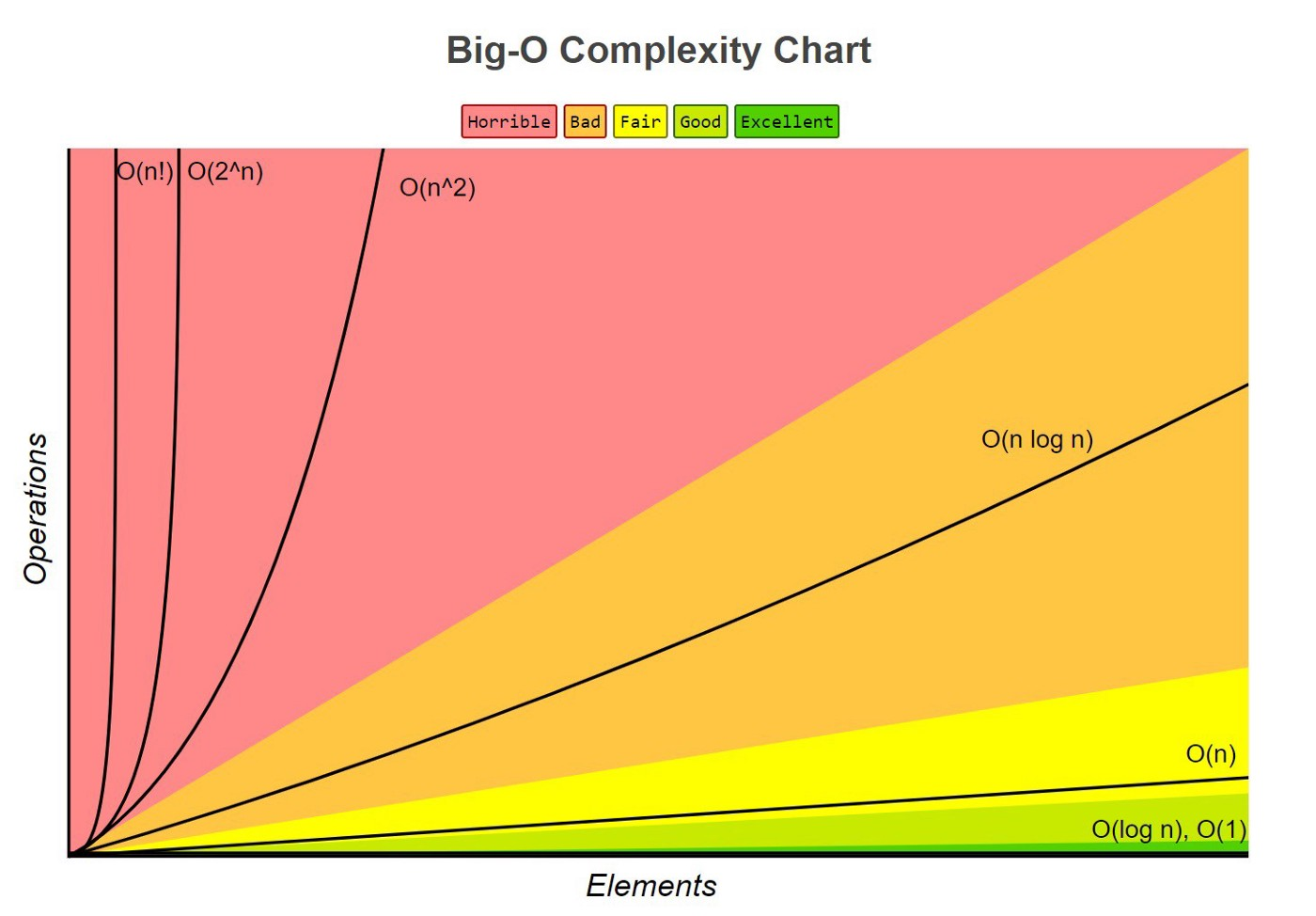# Sorting Algorithms

Since I’m learning algorithms these days (cramming Leetcode actually), I find it quite necessary and interesting to do some notes about sorting algorithms, a series of common knowledge for every programmer.

I’ll be realizing these algorithms myself using Python, in order to put the process in practice for better familiarity.

And here is the GitHub repo for these algorithms.

# Quicksort

""" self-realized quicksort python version """

def partition(l: list, start: int, end: int, reverse: bool = False):
# last element as pivot
pivot = l[end]
pIndex = start
for i in range(start, end):
if reverse:

if l[i] >= pivot:
# swap larger ones to left of the pIndex
l[pIndex], l[i] = l[i], l[pIndex]
pIndex += 1
else:

if l[i] <= pivot:
# swap smaller ones to left of the pIndex
l[pIndex], l[i] = l[i], l[pIndex]
pIndex += 1
# swap the pivot
l[pIndex], l[end] = l[end], l[pIndex]
return pIndex

def quicksort(l: list, start: int, end: int):

if start >= end:
return
pIndex = partition(l, start, end)
quicksort(l, start, pIndex-1)
quicksort(l, pIndex+1, end)

c = [2, 1, 3, 4, 8, 10, 7, 6]
quicksort(c, 0, len(c)-1)
print(c)


Quicksort is a Divide and Conquer algorithm (just like Mergesort). It implements a technique called partition to sort everything. Partition basically uses a pivot (could be any index, I used the last index of every array), and put elements that are lesser to the left of it and larger to the right of it (during a ascending sort).

Then, it uses the [start, pivot_index - 1] and [pivot_index + 1, end] as the array to be sort into the recursive children functions. The algorithm uses index to identify the array and thus it is a in-place (does not create extra data structure to run the algorithm) algorithm.

It is also a non-stable algorithm (a sorting algorithm is said to be stable if it maintains the relative order of records in the case of equality of keys).

It’s time complexity is $O(nlogn)$Referenced from Towards Data Science

# Mergesort

""" self-realized mergesort python version """

def merge(a: list, b: list) -> list:
merged = []
ai, bi = 0, 0
while ai < len(a) and bi < len(b):
if a[ai] <= b[bi]:
merged.append(a[ai])
ai += 1
else:
merged.append(b[bi])
bi += 1

# put in the remaining elements
for i in range(ai, len(a)):
merged.append(a[i])
for i in range(bi, len(b)):
merged.append(b[i])

return merged

def mergesort(l: list):
mid = len(l) // 2

# split in half
a = l[:mid]
b = l[mid:]
if len(l) <= 2:
return merge(a, b)

# recursively merge two split arrays
return merge(mergesort(a), mergesort(b))

c = [2, 4, 1, 6, 8, 5, 7, 7, 3, 7]
print(mergesort(c))


Mergesort is also a Divide and Conquer algorithm, much like the quicksort. It is more intuitive and closer to the divide and conquer nature: it splits the list in half recursively, and merge them back in ascending order.

The merge and sort are divided into two parts, much like the partition and sort in quicksort.

Mergesort is a stable sorting algorithm.
Mergesort’s time complexity: $O(nlogn)$

# Insertion sort

""" self-realized insertion sort python version """

def insertionsort(a: list):
bound = 1
while bound < len(a):
curr, insert = a[bound], 0
# iterate downward from the boundary between sorted and unsorted (bound)
for j in range(bound-1, -1, -1):
if a[j] >= curr:
# move the cards
a[j+1] = a[j]
else:
# found the insertion index
insert = j + 1
break
a[insert] = curr
bound += 1
return a

l = [7, 2, 4, 1, 5, 3]
print(insertionsort(l))


Insertion sort sorts the numbers just like how we human sort cards. It has two parts: sorted and unsorted. And it moves the card from unsorted to sorted by comparing the current unsorted card with each card from the sorted pile, and decide the right index to insert it.

Time complexity: $O(n^2)$
Stability: True

# Bubble sort

""" self-realized bubble sort python version """

def bubblesort(a: list):
length = len(a)
for i in range(length):
flag = 0
for j in range(length - i - 1):
# no need to go through the sorted part
if a[j] >= a[j + 1]:
a[j], a[j + 1] = a[j + 1], a[j]
flag = 1
if flag == 0:
# no swap at all: already sorted
break
return a

l = [2, 3, 1, 4, 7, 5]
print(bubblesort(l))


Bubble sort’s princple is quite straight-foward, it swaps each element with the element right next to it to “bubble up” the larger ones to the right of the array. And it does this process n times to make sure the whole array is sorted.

Time complexity: $O(n^2)$
Stability: True

# Selection sort

""" self-realized selection sort python version """

def find_min_index(a: list, start: int) -> int:
min_ = float('inf')
min_i = 0
for i in range(start, len(a)):
if a[i] <= min_:
min_ = a[i]
min_i = i
return min_i

def selectionsort(a: list) -> list:
count = 0

while count < len(a):
min_i = find_min_index(a, count)
a[count], a[min_i] = a[min_i], a[count]
count += 1

return a

print(selectionsort([2, 3, 1, 4, 7, 5]))


Selection sort turns out to be the easiest sorting algorithm among these ones. Its logic cannot get simpler, it finds the minimum element from the unsorted pile and put the element into the sorted pile. And it repeats this process n times. It is intuitive and I think there are many of us sort cards using this idea.

Time complexity: $O(n^2)$
Stability: False

Tags:

Categories:

Updated: